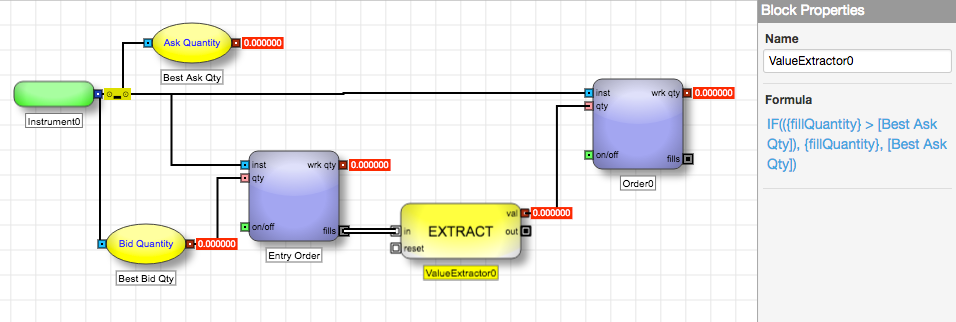When creating a formula, you typically typically type the formula into the formula field, using the components shown in the dialog. For variables originating from other blocks on the canvas or from the content of an input message, you can choose to add them from the drop-down menus. For this task, you will create a formula that exports the fill quantity if the inside market quantity is greater than the fill quantity; otherwise, it exports the available inside market quantity.

To create a formula:

1. From an ADL block that supports formulas (the ValueExtractor block, in this case), click the Forumla edit link in the Block Properties panel.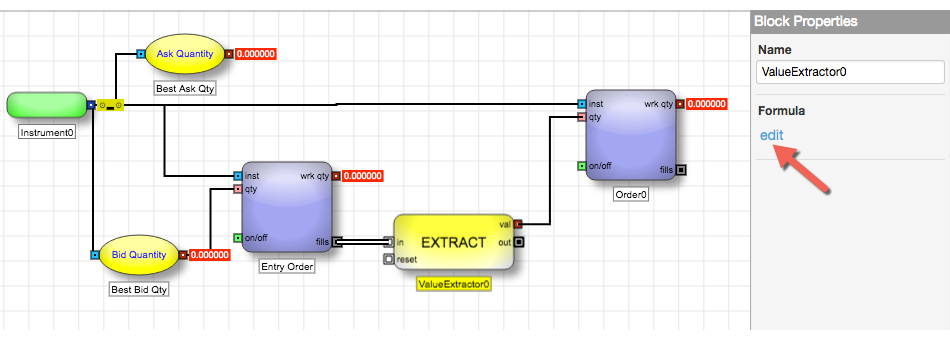The Formula Editor opens.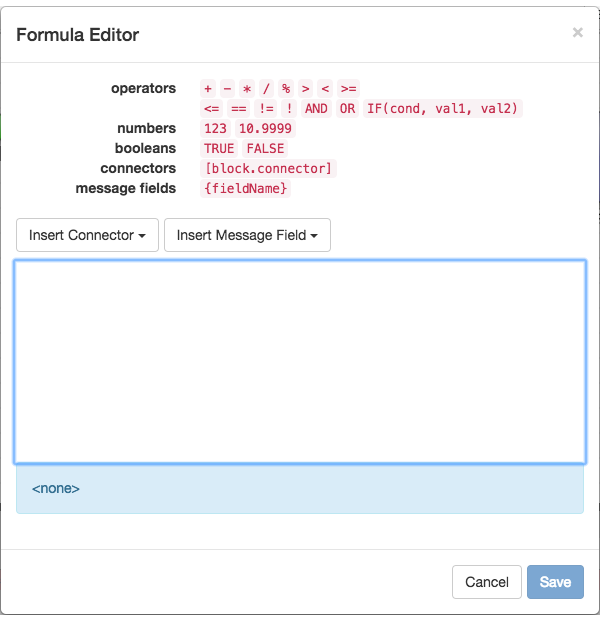2. Begin typing the formula.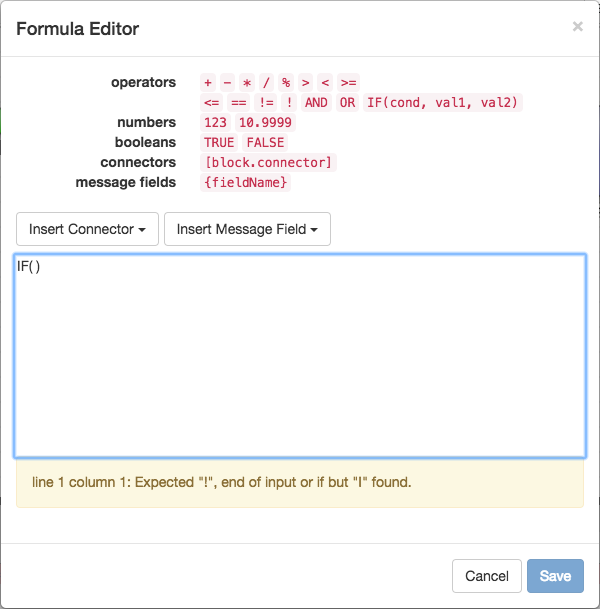Notice the message under the formula field with the yellow background, indicating the formula is not yet valid.

3. In this case, add a value from the input message by selecting it from the Insert Message Field drop-down.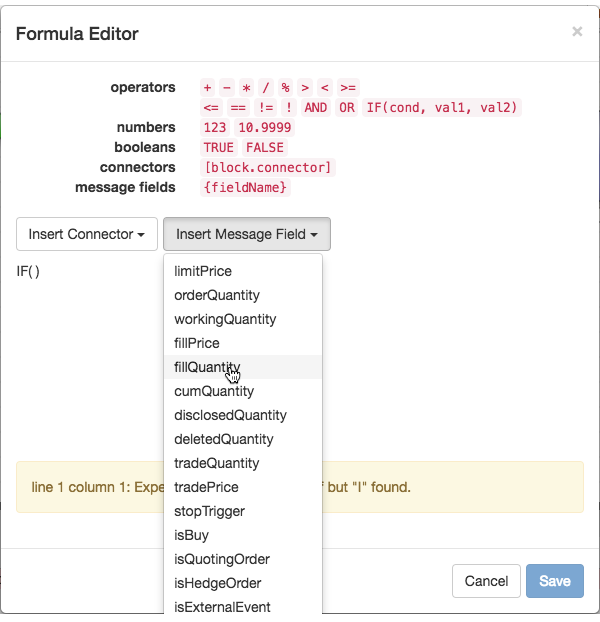A graphic representation of the field is added to the formula. Alternatively, you could have typed {fillQuantity}.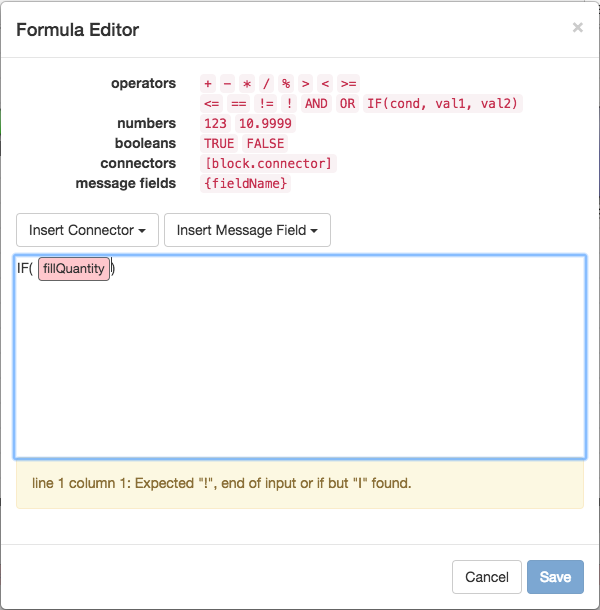4. Type > and then select the value of the Best Ask Qty block from the Insert Connector drop-down.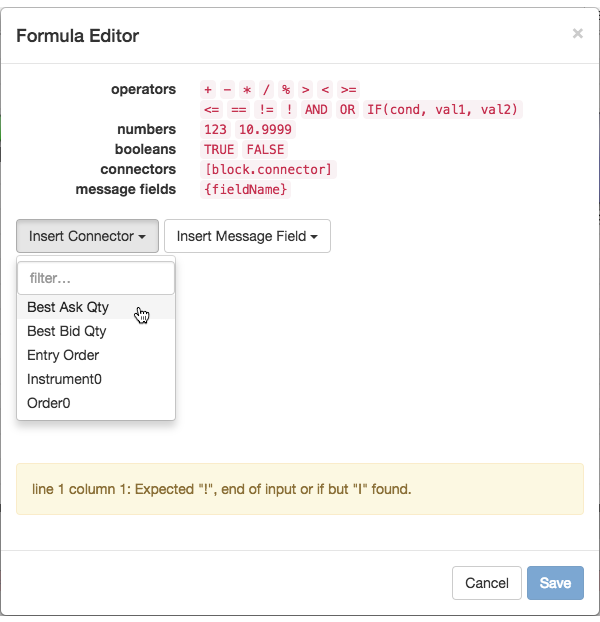A graphic representation of the field is added to the formula. Alternatively, you could have typed [fillQuantity].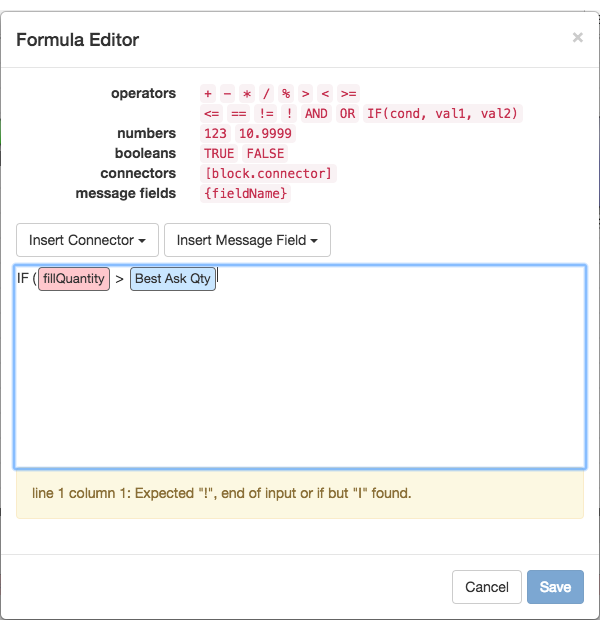5. Enter the remainder of the formula so that it matches the format of the IF(cond, val1, val2) statement.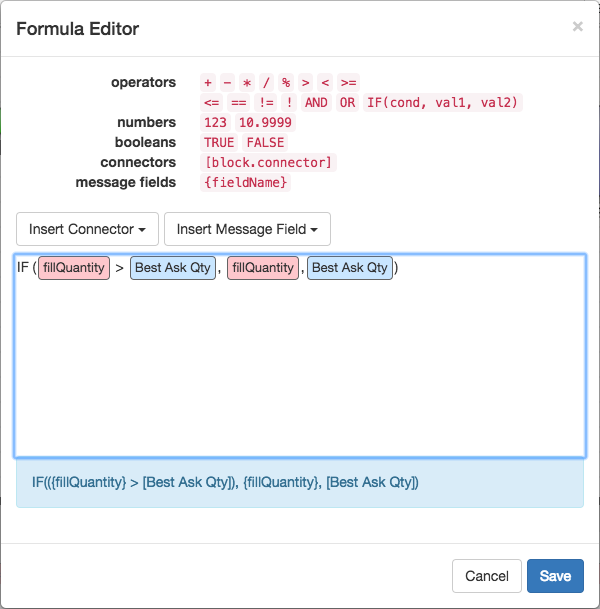Notice the message under the formula field now has a blue background with the valid formula.

6. When finished, click Save.

Notice the formula is displayed in the Block Properties panel.